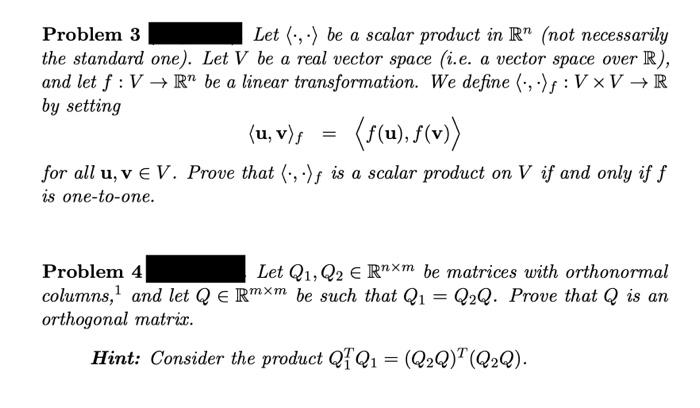# Question Problem 3 Let $$\langle\cdot, \cdot\rangle$$ be a scalar product in $$\mathbb{R}^{n}$$ (not necessarily the standard one). Let $$V$$ be a real vector space (i.e. a vector space over $$\mathbb{R}$$ ), and let $$f: V \rightarrow \mathbb{R}^{n}$$ be a linear transformation. We define $$\langle\cdot, \cdot\rangle_{f}: V \times V \rightarrow \mathbb{R}$$ by setting $\langle\mathbf{u}, \mathbf{v}\rangle_{f}=\langle f(\mathbf{u}), f(\mathbf{v})\rangle$ for all $$\mathbf{u}, \mathbf{v} \in V$$. Prove that $$\langle\cdot, \cdot\rangle_{f}$$ is a scalar product on $$V$$ if and only if $$f$$ is one-to-one. Problem $$4 \quad$$ Let $$Q_{1}, Q_{2} \in \mathbb{R}^{n \times m}$$ be matrices with orthonormal columns, $${ }^{1}$$ and let $$Q \in \mathbb{R}^{m \times m}$$ be such that $$Q_{1}=Q_{2} Q$$. Prove that $$Q$$ is an orthogonal matrix. Hint: Consider the product $$Q_{1}^{T} Q_{1}=\left(Q_{2} Q\right)^{T}\left(Q_{2} Q\right)$$.Transcribed Image Text: Problem 3 Let $$\langle\cdot, \cdot\rangle$$ be a scalar product in $$\mathbb{R}^{n}$$ (not necessarily the standard one). Let $$V$$ be a real vector space (i.e. a vector space over $$\mathbb{R}$$ ), and let $$f: V \rightarrow \mathbb{R}^{n}$$ be a linear transformation. We define $$\langle\cdot, \cdot\rangle_{f}: V \times V \rightarrow \mathbb{R}$$ by setting $\langle\mathbf{u}, \mathbf{v}\rangle_{f}=\langle f(\mathbf{u}), f(\mathbf{v})\rangle$ for all $$\mathbf{u}, \mathbf{v} \in V$$. Prove that $$\langle\cdot, \cdot\rangle_{f}$$ is a scalar product on $$V$$ if and only if $$f$$ is one-to-one. Problem $$4 \quad$$ Let $$Q_{1}, Q_{2} \in \mathbb{R}^{n \times m}$$ be matrices with orthonormal columns, $${ }^{1}$$ and let $$Q \in \mathbb{R}^{m \times m}$$ be such that $$Q_{1}=Q_{2} Q$$. Prove that $$Q$$ is an orthogonal matrix. Hint: Consider the product $$Q_{1}^{T} Q_{1}=\left(Q_{2} Q\right)^{T}\left(Q_{2} Q\right)$$.
Transcribed Image Text: Problem 3 Let $$\langle\cdot, \cdot\rangle$$ be a scalar product in $$\mathbb{R}^{n}$$ (not necessarily the standard one). Let $$V$$ be a real vector space (i.e. a vector space over $$\mathbb{R}$$ ), and let $$f: V \rightarrow \mathbb{R}^{n}$$ be a linear transformation. We define $$\langle\cdot, \cdot\rangle_{f}: V \times V \rightarrow \mathbb{R}$$ by setting $\langle\mathbf{u}, \mathbf{v}\rangle_{f}=\langle f(\mathbf{u}), f(\mathbf{v})\rangle$ for all $$\mathbf{u}, \mathbf{v} \in V$$. Prove that $$\langle\cdot, \cdot\rangle_{f}$$ is a scalar product on $$V$$ if and only if $$f$$ is one-to-one. Problem $$4 \quad$$ Let $$Q_{1}, Q_{2} \in \mathbb{R}^{n \times m}$$ be matrices with orthonormal columns, $${ }^{1}$$ and let $$Q \in \mathbb{R}^{m \times m}$$ be such that $$Q_{1}=Q_{2} Q$$. Prove that $$Q$$ is an orthogonal matrix. Hint: Consider the product $$Q_{1}^{T} Q_{1}=\left(Q_{2} Q\right)^{T}\left(Q_{2} Q\right)$$.&#12304;General guidance&#12305;The answer provided below has been developed in a clear step by step manner.Step1/2First, we prove that if $$\mathrm{{\left(,\right)}}$$ is a scalar product on $$\mathrm{{V}}$$, then $$\mathrm{{f}}$$ is one-to-one.Suppose that $$\mathrm{{\left(,\right)}}$$ is a scalar product on $$\mathrm{{V},}$$ is not one-to-one. Then there exist vectors $$\mathrm{{u}_{{1}}{u}_{{2}}}$$ in V such that . Then we have$$\mathrm{{\left({u}_{{1}}-{u}_{{2}},{u}_{{1}}-{u}_{{2}}\right)}={\left({u}_{{1}},{u}_{{1}}\right)}-{2}{\left({u}_{{1}},{u}_{{2}},\right)}+{\left({u}_{{2}},{u}_{{2}}\right)}>{0}}$$Since $$\mathrm{{\left(,\right)}}$$ is a positive definite scalar product. On the other hand, we have$$\mathrm{{\left({f{{\left({u}_{{1}}\right)}}}-{f{{\left({u}_{{2}}\right)}}},{f{{\left({u}_{{1}}\right)}}}-{f{{\left({u}_{{2}}\right)}}}\right)}={\left({f{{\left({u}_{{1}}\right)}}},{f{{\left({u}_{{2}}\right)}}}\right)}-{2}{f{{\left({\left({u}_{{1}}\right)},{f{{\left({u}_{{2}}\right)}}}\right)}}}+{\left({f{{\left({u}_{{2}}\right)}}},{f{{\left({u}_{{2}}\right)}}}\right)}}$$$$\mathrm{={\left({u}_{{1}},{u}_{{1}}\right)}-{2}{\left({u}_{{1}},{u}_{{2}}\right)}+{\left({u}_{{2}},{u}_{{2}}\right)}}$$since $$\mathrm{{\left(,\right)}}$$ is induced by f. ExplanationTherefore, we obtain a contradiction, which implies that f must be one-to-one.Next, we prove that if f is one-to-one, then \( \mathrm{{\left(,\right) ... See the full answer